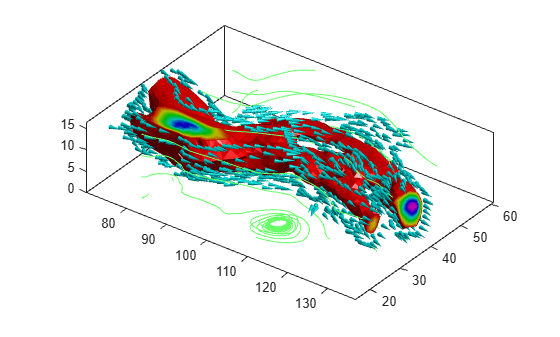# Visualizing Volume Data

This example shows several methods for visualizing volume data in MATLAB®.

### Display Isosurface

An isosurface is a surface where all the points within a volume of space have a constant value. Use the `isosurface` function to generate the faces and vertices for the outside of the surface and the `isocaps` function to generate the faces and vertices for the end caps of the volume. Use the `patch` command to draw the volume and its end caps.

```load mri D D = squeeze(D); limits = [NaN NaN NaN NaN NaN 10]```
```limits = 1×6 NaN NaN NaN NaN NaN 10 ```
```[x, y, z, D] = subvolume(D, limits); [fo,vo] = isosurface(x,y,z,D,5); [fe,ve,ce] = isocaps(x,y,z,D,5); figure p1 = patch('Faces', fo, 'Vertices', vo); p1.FaceColor = 'red'```
```p1 = Patch with properties: FaceColor: [1 0 0] FaceAlpha: 1 EdgeColor: [0 0 0] LineStyle: '-' Faces: [23351x3 double] Vertices: [12406x3 double] Show all properties ```
`p1.EdgeColor = 'none'`
```p1 = Patch with properties: FaceColor: [1 0 0] FaceAlpha: 1 EdgeColor: 'none' LineStyle: '-' Faces: [23351x3 double] Vertices: [12406x3 double] Show all properties ```
```p2 = patch('Faces', fe, 'Vertices', ve, ... 'FaceVertexCData', ce)```
```p2 = Patch with properties: FaceColor: [0 0 0] FaceAlpha: 1 EdgeColor: [0 0 0] LineStyle: '-' Faces: [27265x3 double] Vertices: [14250x3 double] Show all properties ```
```p2.FaceColor = 'interp'; p2.EdgeColor = 'none'; view(-40,24) daspect([1 1 0.3]) colormap(gray(100)) box on camlight(40,40) camlight(-20,-10) lighting gouraud```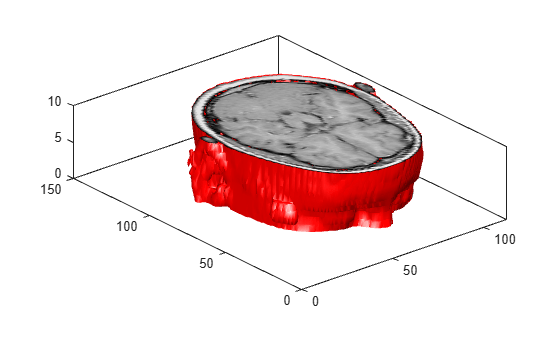### Create Cone Plot

The `coneplot` command plots velocity vectors as cones at x, y, z points in a volume. The cones represent the magnitude and direction of the vector field at each point.

```cla load wind u v w x y z [m,n,p] = size(u)```
```m = 35 ```
```n = 41 ```
```p = 15 ```
```[Cx, Cy, Cz] = meshgrid(1:4:m,1:4:n,1:4:p); h = coneplot(u,v,w,Cx,Cy,Cz,y,4); set(h,'EdgeColor', 'none') axis tight equal view(37,32) box on colormap(hsv) light```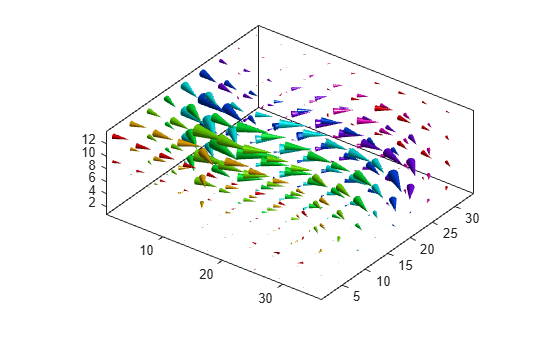### Plot Streamlines

The `streamline` function plots streamlines for a velocity vector at x, y, z points in a volume to illustrate the flow of a 3-D vector field.

```cla [m,n,p] = size(u); [Sx, Sy, Sz] = meshgrid(1,1:5:n,1:5:p); streamline(u,v,w,Sx,Sy,Sz) axis tight equal view(37,32) box on```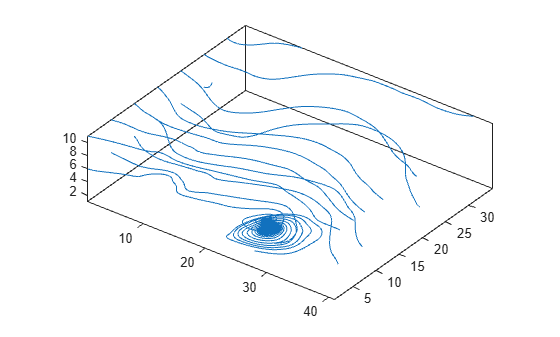### Plot Streamtubes

The `streamtube` function plots streamtubes for a velocity vector at x, y, z points in a volume. The width of the tube is proportional to the normalized divergence of the vector field at each point.

```cla [~,n,p] = size(u); [Sx, Sy, Sz] = meshgrid(1,1:5:n,1:5:p); h = streamtube(u,v,w,Sx,Sy,Sz); set(h, 'FaceColor', 'cyan') set(h, 'EdgeColor', 'none') axis tight equal view(37,32) box on light```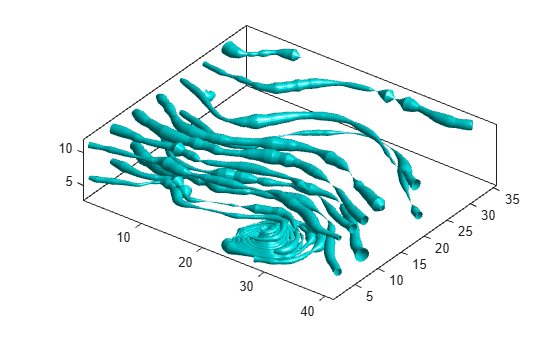### Combine Volume Visualizations

Combine volume visualization in a single plot to get a more comprehensive picture of a velocity field within a volume.

```cla spd = sqrt(u.*u + v.*v + w.*w); [fo,vo] = isosurface(x,y,z,spd,40); [fe,ve,ce] = isocaps(x,y,z,spd,40); p1 = patch('Faces', fo, 'Vertices', vo); p1.FaceColor = 'red'```
```p1 = Patch with properties: FaceColor: [1 0 0] FaceAlpha: 1 EdgeColor: [0 0 0] LineStyle: '-' Faces: [5340x3 double] Vertices: [2727x3 double] Show all properties ```
`p1.EdgeColor = 'none'`
```p1 = Patch with properties: FaceColor: [1 0 0] FaceAlpha: 1 EdgeColor: 'none' LineStyle: '-' Faces: [5340x3 double] Vertices: [2727x3 double] Show all properties ```
```p2 = patch('Faces', fe, 'Vertices', ve, ... 'FaceVertexCData', ce)```
```p2 = Patch with properties: FaceColor: [0 0 0] FaceAlpha: 1 EdgeColor: [0 0 0] LineStyle: '-' Faces: [464x3 double] Vertices: [301x3 double] Show all properties ```
`p2.FaceColor = 'interp'`
```p2 = Patch with properties: FaceColor: 'interp' FaceAlpha: 1 EdgeColor: [0 0 0] LineStyle: '-' Faces: [464x3 double] Vertices: [301x3 double] Show all properties ```
`p2.EdgeColor = 'none' `
```p2 = Patch with properties: FaceColor: 'interp' FaceAlpha: 1 EdgeColor: 'none' LineStyle: '-' Faces: [464x3 double] Vertices: [301x3 double] Show all properties ```
```[fc, vc] = isosurface(x, y, z, spd, 30); [fc, vc] = reducepatch(fc, vc, 0.2); h1 = coneplot(x,y,z,u,v,w,vc(:,1),vc(:,2),vc(:,3),3); h1.FaceColor = 'cyan'; h1.EdgeColor = 'none'; [sx, sy, sz] = meshgrid(80, 20:10:50, 0:5:15); h2 = streamline(x,y,z,u,v,w,sx,sy,sz); set(h2, 'Color', [.4 1 .4]) axis tight equal view(37,32) box on light```